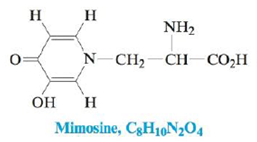Chapter 22, Problem 49E

Chapter
Section
Textbook Problem

# Mimosine is a natural product found in large quantities in the seeds and foliage of some legume plants and has been shown to cause inhibition of hair growth and hair loss in mice.a. What functional groups are present in mimosine?b. Give the hybridization of the eight carbon atoms in mimosine.c. How many σ and π bonds are found in mimosine?

(a)

Interpretation Introduction

Interpretation: The given questions based upon the structure of mimosine are to be answered.

Concept introduction: A functional group is a group of elements that changes the physical as well as chemical properties of a compound. They play a significant role in naming and in reactivity of a chemical reaction. Some of the common examples of functional groups are alcohol, carboxylic acid, amine, aldehyde, ketone and many others.

Explanation

Explanation

To determine: The functional groups present in mimosine.

Functional groups present in mimosine are ketone, alcohol, amine and carboxylic acid.

Ketones are characterized by the structure R(CO)R' . Since the given compound contains C=O bond, therefore the given compound has ketone functional group.

Alcohols are characterized by the structure R-OH

(b)

Interpretation Introduction

Interpretation: The given questions based upon the structure of mimosine are to be answered.

Concept introduction: A functional group is a group of elements that changes the physical as well as chemical properties of a compound. They play a significant role in naming and in reactivity of a chemical reaction. Some of the common examples of functional groups are alcohol, carboxylic acid, amine, aldehyde, ketone and many others.

(c)

Interpretation Introduction

Interpretation: The given questions based upon the structure of mimosine are to be answered.

Concept introduction: A functional group is a group of elements that changes the physical as well as chemical properties of a compound. They play a significant role in naming and in reactivity of a chemical reaction. Some of the common examples of functional groups are alcohol, carboxylic acid, amine, aldehyde, ketone and many others.

### Still sussing out bartleby?

Check out a sample textbook solution.

See a sample solution

#### The Solution to Your Study Problems

Bartleby provides explanations to thousands of textbook problems written by our experts, many with advanced degrees!

Get Started